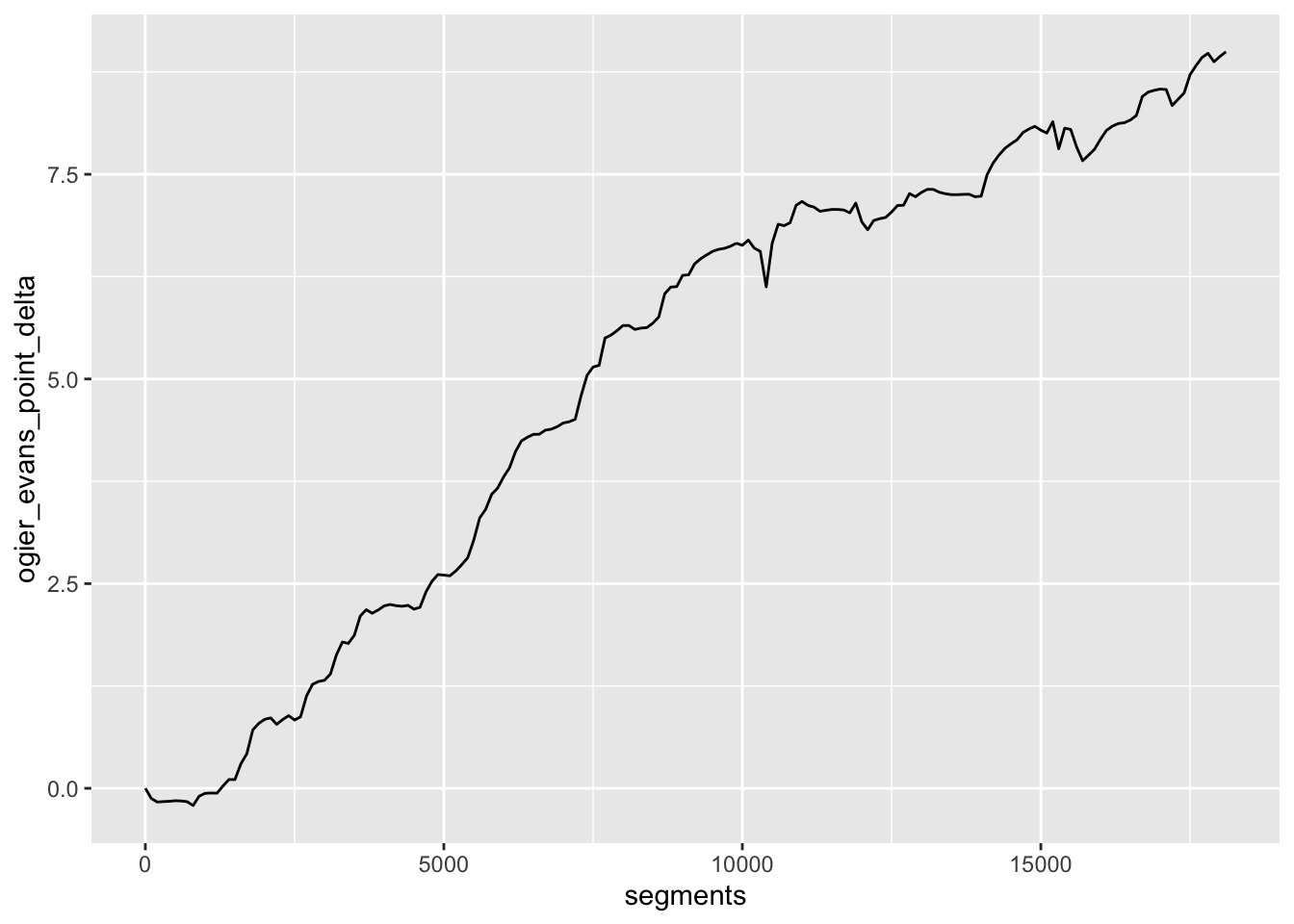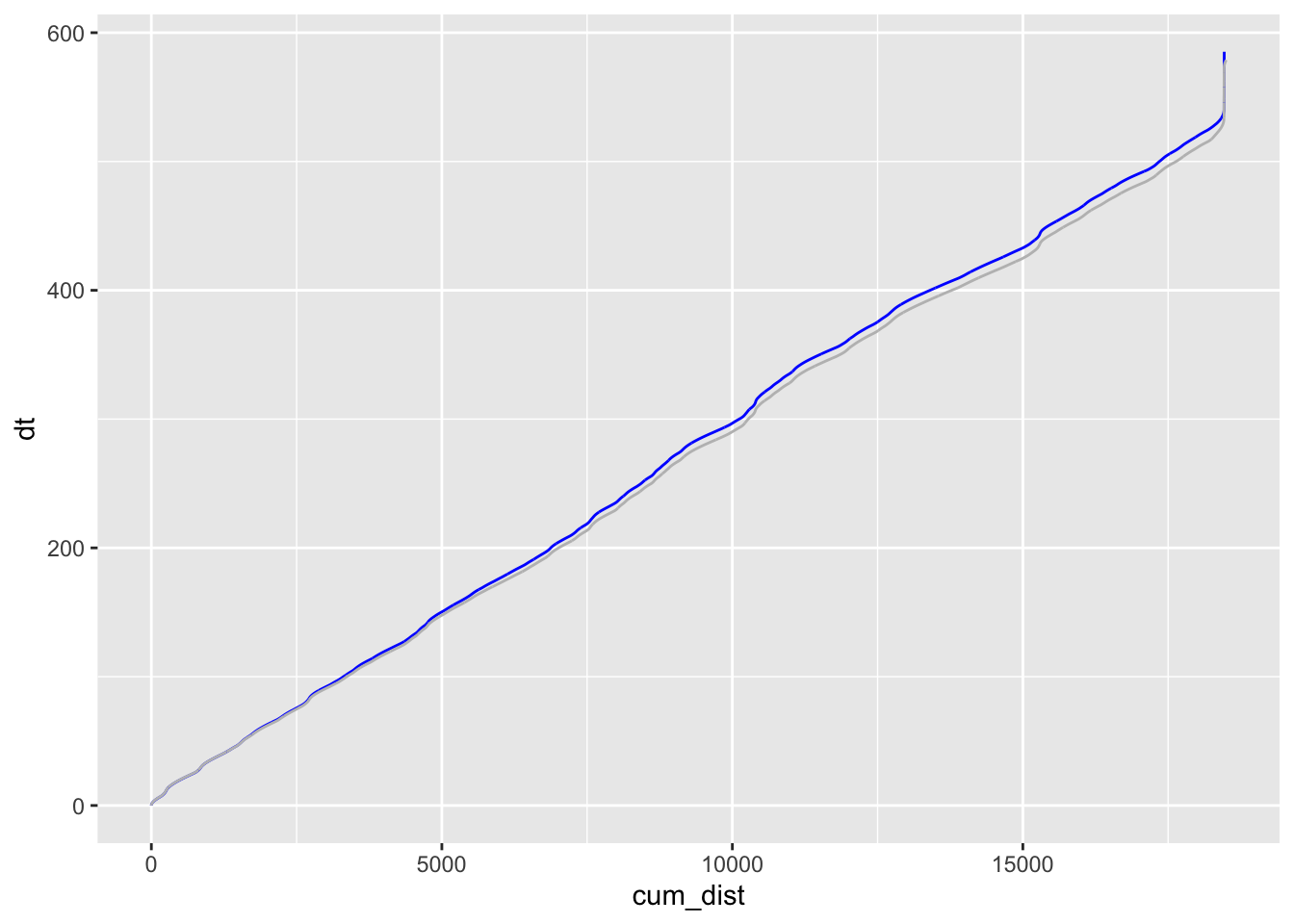# 15 Comparing Times Into Stage

How do our drivers compare based on the time it takes them to driver a particular distance into the stage?

Recall that we are using each car’s own accumulated distance in to stage measure, rather than comparing times at distances into stage measured along some common, idealised route.

Sample common points to find the difference:

``````segment_length=100

# stage_length set in earlier section
segments = seq(from=0, to=stage_length, by = segment_length)

ogier_full_estimator = approxfun(trj_ogier\$cum_dist,
trj_ogier\$displacementTime)``````
``````## Warning in regularize.values(x, y, ties, missing(ties), na.rm = na.rm):
## collapsing to unique 'x' values``````
``````evans_full_estimator = approxfun(trj_evans\$cum_dist,
trj_evans\$displacementTime)``````
``````## Warning in regularize.values(x, y, ties, missing(ties), na.rm = na.rm):
## collapsing to unique 'x' values``````
``````ogier_time_points = ogier_full_estimator(segments)
evans_time_points = evans_full_estimator(segments)

ogier_evans_point_delta = ogier_time_points - evans_time_points

# Difference in time take to reach a particular point in the stage
ggplot()+geom_line(aes(x=segments, y=ogier_evans_point_delta))``````Compare time into stage for a given distance into stage. Note that this is the distance into the stage as calculated from the trajectory, rather than by comparison of distances into the same (idealised) route.

``````ggplot() +
geom_line(data=(trj_ogier %>% mutate(dt=as.double(displacementTime)) %>% filter(dt >=0)),
aes(x=cum_dist,y=dt), color='blue') +
geom_line(data=(trj_evans %>% mutate(dt=as.double(displacementTime)) %>% filter(dt >=0)),
aes(x=cum_dist,y=dt), color='grey') ``````